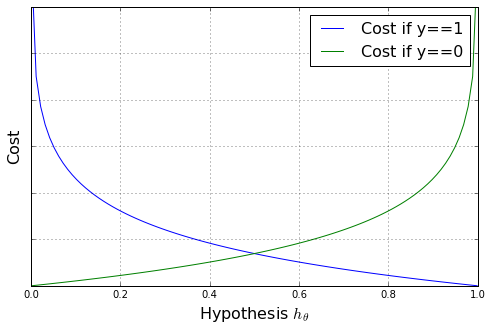# Fundamental Concept Review - Regression vs. Classification

### Do you know the difference?

Posted by David Kaleko on Mon 23 October 2017

Many people new to machine learning start doing some research and quickly learn to ask one of the most important fundamental questions to understand about supervised learning problems:

### What's the difference between regression and classification?¶

Regression: the output variable takes continuous values.
Classification: the output variable takes class labels.


Great! We're done. Shortest blog post ever!

Just kidding.

While that stackexchange answer is indeed true, and is indeed generally a sufficient explanation for someone in perhaps a managerial or executive role, it is most definitely insufficient for someone working closey with machine learning.

It turns out, both regression and classification problems end up computing continuous values. Why then can't we take a regression problem, slap a threshold on the output (i.e. all predictions above 50 are labeled "1" and all predictions below 50 are labeled "0"), and call it a classification problem? Regression problems and classification problems are fundamentally different beasts.

### Minimizing Cost Functions¶

Both regression and classification algorithms are at their core minimizing a cost function, $J$. They do this by taking some training data, forming a hypothesized predicted value $h_\theta$ (where $\theta$ represents the parameters that define the model) for each point, and determining how wrong that prediction is by comparing it to a true label, $y$. How wrong the prediction was is called the cost.

Take for example the case of basic linear regression: the cost is the summed squared difference between $h_\theta$ and $y$ for all $m$ training points. If the value the model predicts is far from the true value in the training set, the cost is high; if the model predicts the value perfectly, the cost is low (zero).The model tries one set of parameters $\theta$, computes the cost, then tries another set of parameters, computes the cost, and continues until it finds the set of parameters that result in the smallest cost (algorithms generally do this parameter tuning in a smart way, often via Gradient Descent).

### Regression vs. Classification¶

Now we can start talking about regression vs. classification problems. For a quick clarification, here we talk about binary classification problems, where the label $y$ can be only 0 or 1 (multi-class classification problems are binary classification problems at their core).

In a regression problem, the hypothesis $h_\theta$ can be any real valued number, from negative infinity to infinity. The labels, $y$ are similarly real valued. The cost function is just comparing $h_\theta$ to $y$ in some way (i.e. squared difference).

In a classification problem, the hypothesis is still real valued, but forced to be between 0 and 1. It's generally forced this way by passing a fully real-valued hypothesis through a sigmoid function. What about the cost function for a binary classification problem?

The cost function for a classification problem changes depending on the true label.

Ding ding ding! This is the fundamental difference between regression and binary classification problems. In a binary classification problem, the cost function when the label is 1 is something like $-log(h_\theta)$ while when the label is 0 it's something like $-log(1-h_\theta)$. That is, if the true label $y$ is 1, the cost is zero (good!) if the prediction, $h_\theta$ is 1. However, the cost goes to infinity the farther from 1 (closer to zero) $h_\theta$ is. The opposite is true if the true label $y$ is 0. This is what constitutes the fundamental difference between regression and classification problems.In summary, regression and binary classification problems are fundamentally different. Regression problems output continuous hypotheses, and classification problems output discrete ones. More subtly, the cost minimization is performed differently between a regression and a classification problem, so a classification problem is not just a regression problem with a threshold applied on the outputs!